# Mechanics of Materials: Strain

### Strain

So far, we've focused on the stress within structural elements. When you apply stress to an object, it deforms. Think of a rubber band: you pull on it, and it gets longer – it stretches. Deformation is a measure of how much an object is stretched, and strain is the ratio between the deformation and the original length.  Think of strain as percent elongation – how much bigger (or smaller) is the object upon loading it.

Just like stress, there are two types of strain that a structure can experience: 1. Normal Strain and 2. Shear Strain. When a force acts perpendicular (or "normal") to the surface of an object, it exerts a normal stress.  When a force acts parallel to the surface of an object, it exerts a shear stress.

Let's consider a rod under uniaxial tension. The rod elongates under this tension to a new length, and the normal strain is a ratio of this small deformation to the rod's original length.Strain is a unitless measure of how much an object gets bigger or smaller from an applied load. Normal strain occurs when the elongation of an object is in response to a normal stress (i.e. perpendicular to a surface), and is denoted by the Greek letter epsilon. A positive value corresponds to a tensile strain, while negative is compressive. Shear strain occurs when the deformation of an object is response to a shear stress (i.e. parallel to a surface), and is denoted by the Greek letter gamma.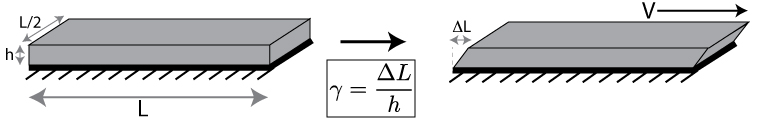### Mechanical Behavior of Materials

Clearly, stress and strain are related. Stress and strain are related by a constitutive law, and we can determine their relationship experimentally by measuring how much stress is required to stretch a material. This measurement can be done using a tensile test.  In the simplest case, the more you pull on an object, the more it deforms, and for small values of strain this relationship is linear. This linear, elastic relationship between stress and strain is known as Hooke's Law. If you plot stress versus strain, for small strains this graph will be linear, and the slope of the line will be a property of the material known as Young's Elastic Modulus. This value can vary greatly from 1 kPa for Jello to 100 GPa for steel.  For most engineering materials, the linear region of the stress-strain diagram only occurs for very small strains (<0.1%). In this course, we will focus only on materials that are linear elastic (i.e. they follow Hooke's law) and isotropic (they behave the same no matter which direction you pull on them).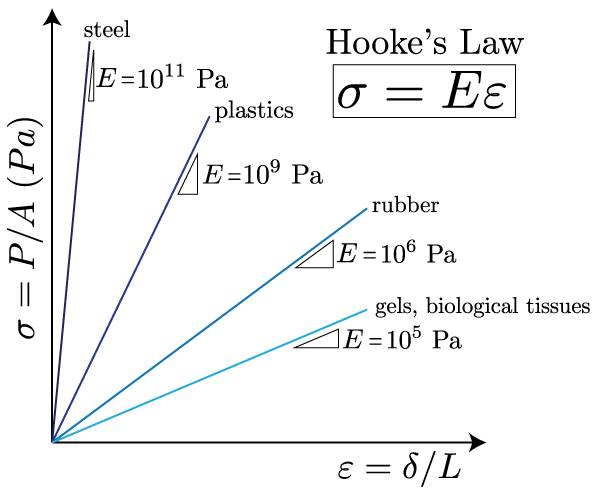From Hooke's law and our definitions of stress and strain, we can easily get a simple relationship for the deformation of a material.Intuitively, this exam makes a bit of sense: apply more load, get a larger deformation; apply the same load to a stiffer or thicker material, get less deformation. If the structure changes shape, or material, or is loaded differently at various points, then we can split up these multiple loadings using the principle of superposition## Generalized Hooke's Law

In the last lesson, we began to learn about how stress and strain are related – through Hooke's law. But, up until this point we've only considered a very simplified version of Hooke's law: we've only talked about stress or strain in one direction.  In this lesson, we're going to consider the generalized Hooke's law for homogenous, isotropic, and elastic materials being exposed to forces on more than one axis.

First things first, even just pulling (or pushing) on most materials in one direction actually causes deformation in all three orthogonal directions. Let's go back to that first illustration of strain. This time, we will account for the fact that pulling on an object axially causes it to compress laterally in the transverse directions: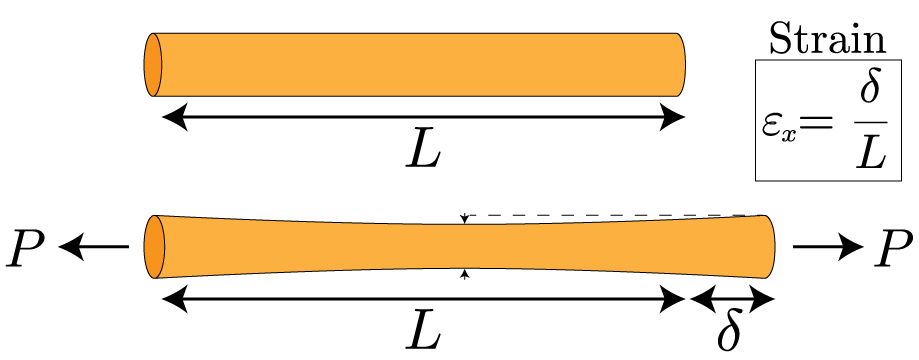So, pulling on it in the x-direction causes it to shrink in the z directions. This property of a material is known as Poisson's ratio, and it is denoted by the Greek letter nu, and is defined as:Or, more mathematically, using the axial load shown in the above image, we can write this out as an equation: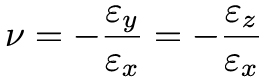Since Poisson's ratio is a ratio of two strains, and strain is dimensionless, Poisson's ratio is also unitless. Poisson's ratio is a material property. Poisson's ratio can range from a value of -1 to 0.5. For most engineering materials, for example steel or aluminum have a Poisson's ratio around 0.3, and rubbers have a Poisson's ratio around 0.5, which are referred to as "incompressible". Incompressible simply means that any amount you compress it in one direction, it will expand the same amount in it's other directions – hence, its volume will not change.

There has been some very interesting research in the last decade in creating structured materials that utilize geometry and elastic instabilities (a topic we’ll cover briefly in a subsequent lecture) to create auxetic materials – materials with a negative Poisson’s ratio. Physically, this means that when you pull on the material in one direction it expands in all directions (and vice versa):

This principle can be applied in 3D to make expandable/collapsible shells as well:

Through Poisson's ratio, we now have an equation that relates strain in the y or z direction to strain in the z direction. We can in turn relate this back to stress through Hooke's law. This is an important note: pulling on an object in one direction causes stress in only that direction, and causes strain in all three directions. So, sigmay = sigmaz = 0. Let's write out the strains in the y and z direction in terms of the stress in the direction.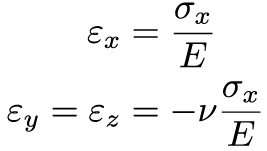Remember, up until this point, we've only considered uniaxial deformation. In reality, structures can be simultaneously loaded in multiple directions, causing stress in those directions. A helpful way to understand this is to imagine a very tiny "cube" of material within an object. That cube can have stresses that are normal to each surface, like this: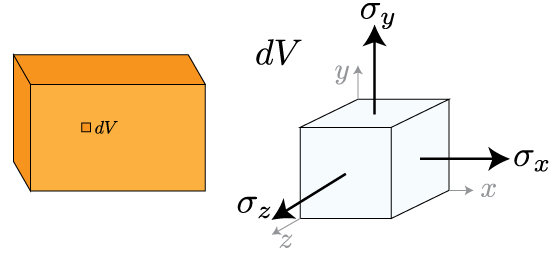So, applying a load in the x direction causes a normal stress in that direction, and the same is true for normal stresses in the y and z directions. And, as we now know, stress in one direction causes strain in all three directions. So now we incorporate this idea into Hooke's law, and write down equations for the strain in each direction as: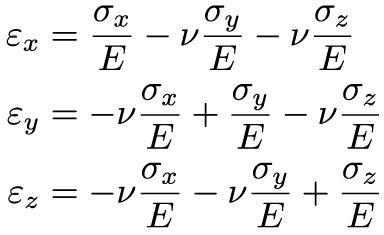These equations look harder than they really are: strain in each direction (or, each component of strain) depends on the normal stress in that direction, and the Poisson's ratio times the strain in the other two directions. Now we have equations for how an object will change shape in three orthogonal directions.  Well, if an object changes shape in all three directions, that means it will change its volume. A simple measure for this volume change can be found by adding up the three normal components of strain:Now that we have an equation for volume change, or dilation, in terms of normal strains, we can rewrite it in terms of normal stresses.A very common type of stress that causes dilation is known as hydrostatic stress. This is just simply a pressure that acts equally on the entire material. Since it is acting equally, that means:So, in the case of hydrostatic pressure we can reduce our final equation for dilation to the following:This final relationship is important, because it is a constitutive relationship for how a material's volume changes under hydrostatic pressure. The prefactor to p can be rewritten as a material's bulk modulusK.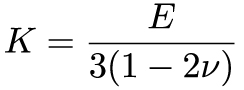Finally, let's get back to the idea of "incompressible" materials. What happens to K – the measure of how a material changes volume under a given pressure – if Poisson's ratio for the material is 0.5?

## Hooke's Law in Shear

In the previous section we developed the relationships between normal stress and normal strain. Now we have to talk about shear. Let's go back to that imaginary cube of material. In addition to external forces causing stresses that are normal to each surface of the cube, the forces can causes stresses that are parallel to each cube face. And, as we know, stresses parallel to a cross section are shear stressesNow that cube of material looks a lot more complicated, but it's really not too bad. On each surface there are two shear stresses, and the subscripts tell you which direction they point in and which surface they are parallel to. For instance, take the right face of the cube. Stresses normal to this face are normal stresses in the x direction. There are two stresses parallel to this surface, one pointing in the y direction (denoted tauxy) and one pointing in the z direction (denoted tauxz). In order for the cube to be in equilibrium, tauxy = tauyx (otherwise, the cube would rotate). Therefore, there are now six stresses (sigmax, sigmay, sigmaz, tauxy, tauyz, tauxz) that characterize the state of stress within a homogenous, isotropic, elastic material.

So, how do these shear stresses relate to shear strains? Hooke's law in shear looks very similar to the equation we saw for normal stress and strain: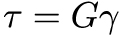In this equation, the proportionality between shear stress and shear strain is known as the shear modulus of a material. That's the equation in its general form, but we can rewrite it more explicitly in terms of its components of x,y, and z. Doing so will give us the generalized Hooke's law for homogenous, isotropic, elastic materials.In our generalized Hooke's law we have our six components of stress and strain, and three material properties. A natural question to as is how do these three material properties relate to each other? That relationship is given by the following equation: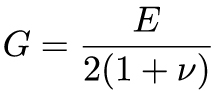## Summary

We've introduced the concept of strain in this lecture. Strain is the deformation of a material from stress. It is simply a ratio of the change in length to the original length. Deformations that are applied perpendicular to the cross section are normal strains, while deformations applied parallel to the cross section are shear strains. For linear, elastic materials, stress is linearly related to strain by Hooke's law. The proportionality of this relationship is known as the material's elastic modulus. Using Hooke's law, we can write down a simple equation that describes how a material deforms under an externally applied load.

Additionally, we learned about multiaxial loading in this section. In particular, we learned that stress in one direction causes deformation in three directions. This occurs due to a material property known as Poisson's ratio –  the ratio between lateral and axial strains. The strains occurring in three orthogonal directions can give us a measure of a material's dilation in response to multiaxial loading. In particular, a material can commonly change volume in response to changes in external pressure, or hydrostatic stress. This lead to a definition of a materials resistance to volume change under hydrostatic stress – the bulk modulus. By inspecting an imaginary cubic element within an arbitrary material, we were able to envision stresses occurring normal and parallel to each cube face. This gave us six stresses and six strains (three normal and three shear) that we related to each other using a generalized Hooke's law for homogenousisotropic, and elastic materials. These components of multiaxial stress and strain are related by three material properties: Young's elastic modulus, the shear modulus, and Poisson's ratio.This material is based upon work supported by the National Science Foundation under Grant No. 1454153. Any opinions, findings, and conclusions or recommendations expressed in this material are those of the author(s) and do not necessarily reflect the views of the National Science Foundation.